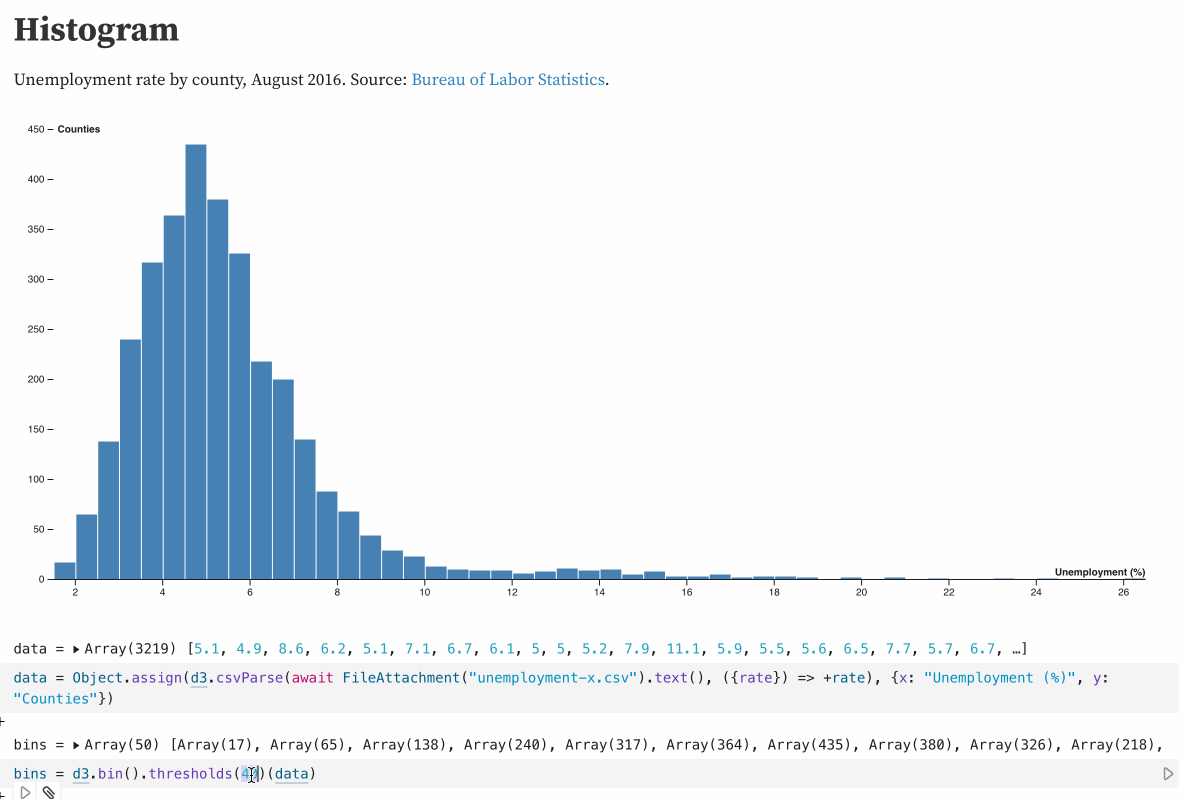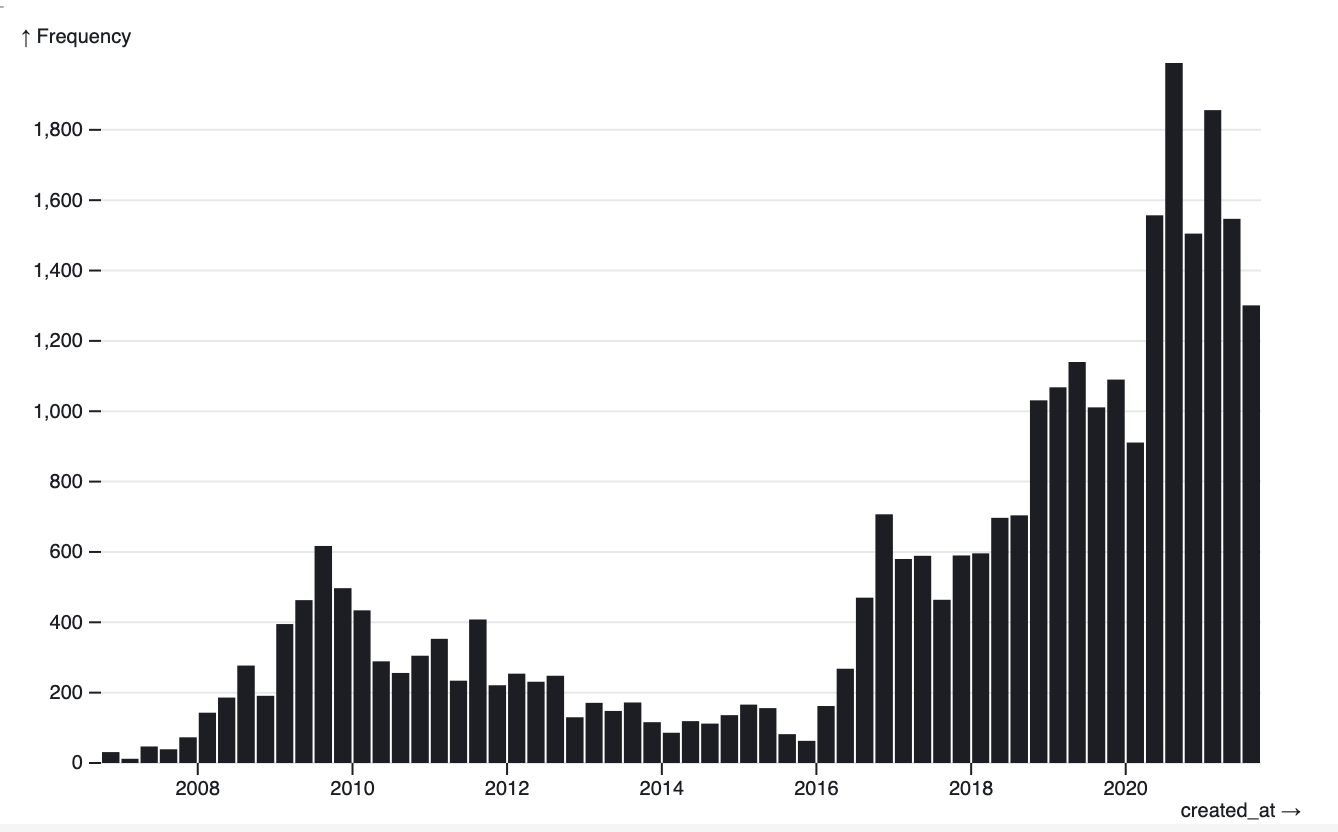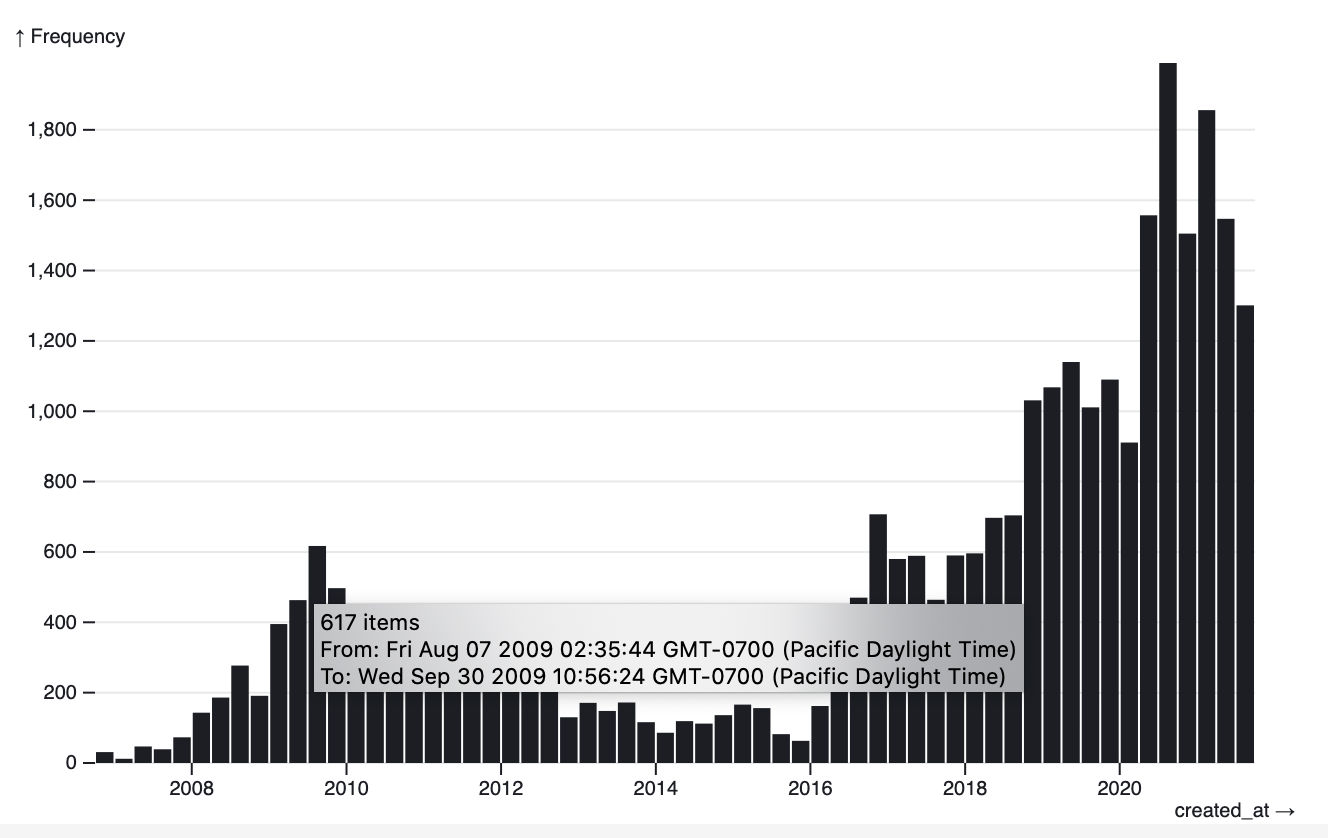# Histogram with tooltips in Observable Plot

Given an array of datetime objects, I wanted to plot a histogram. But I wanted to automatically pick a bucket size for that histogram that resulted in something interesting, no matter what range of time the individual points covered.

I figured out that d3 has a mechanism for this: `d3.bin().thresholds()` (docs here). This defaults to using Sturges' formula but has various other options.

This d3 Histogram notebook helped me figure this out:## Implementing this in Observable Plot

The higher level Observable Plot library provides binning. Here's the recipe I figured out for generating a histogram with that, in my Histogram of my tweets over time notebook:

```Plot.plot({
y: {
grid: true
},
marks: [Plot.rectY(tweets, Plot.binX({ y: "count" }, { x: "created_at" }))]
})```It turns out that while d3 uses Sturges' formula Observable Plot instead uses Scott's rule:

D3 chose Sturges’ formula because it was the popular choice (at the time) but Observable Plot defaults to Scott’s rule, with a max of 200 bins, which tends to perform better. Related: https://robjhyndman.com/papers/sturges.pdf

Mike Bostock - @mbostock

I wanted to add tooltips to the above chart. This was the hardest part to figure out - it turns out that second argument to `.binX()` can take a `title` option, which can be a function that accepts a `bin` array of items and returns a title.

UPDATE 27th January 2022: The below code example no longer works - the `bin` argument passed to the `title:` function used to be a list of items in that bin, but now appears to just be a single item.

This is what I ended up using:

```Plot.plot({
y: {
grid: true
},
marks: [
Plot.rectY(
tweets,
Plot.binX(
{ y: "count" },
{
x: "created_at",
title: bin => {
let sorted = [...Array.from(bin).map(t => t.created_at)].sort();
let min = sorted;
let max = sorted.slice(-1);
let count = sorted.length;
return `\${sorted.length} item\${
sorted.length == 0 ? '' : 's'
}\nFrom: \${min}\nTo: \${max}`;
}
}
)
)
]
})```
• `[...bin.map(t => t.created_at)].sort()` is a recipe for creating a sorted copy of an array of values
• `max = sorted.slice(-1)` gets the last item in that array

Then I compose and return the string.